This sketch will read RMC-data from a GPS receiver and show it on a 4 X 20 LCD display, while converting position information to MAIDENHEAD locator.  Very handy if you are operating /portable during contest, field day etc.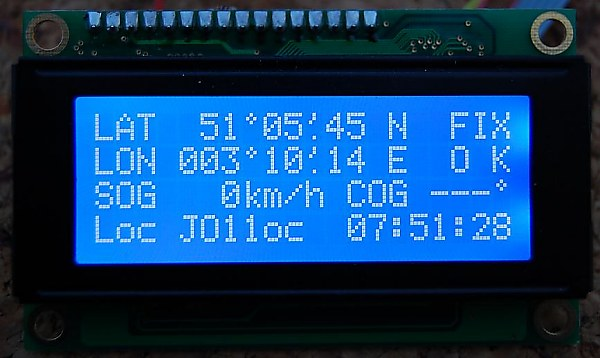In addition, the backlight of the display (which consumes a lot of power) is adjusted according to the ambient light conditions.  To regulate this efficiently with a small transistor (BC547 or equivalent NPN type), the PWM frequency has been lowered to allow clean on/off condition.  The switching transistor is placed in the backlight line which runs to GND, the + of backlight is connected to +5v in series with a 22 ohm current limiting resistor. The modified PWM frequency  implies that  some time related ARDUINO instructions (like DELAY) have arguments adapted accordingly (approx x 3)

The display shows latitude, longitude, fix status ( 'FIX OK' or blinking 'NO FIX'),  Speed over ground (SOG) en course over ground (COG), the Maidenhead locator and the UTC time.  If no serial data from GPS is detected, an alarm message is shown.  Battery condition is tested at startup and each minute about. A small buzzer alerts in case of low battery, no serial data received, or loss of fix. I didn't effectively check if the Maidenhead locator calculation is OK in the southern hemisphere and western longitudes.  Remark : in V2.00 there was a mistake in locator conversion with S or W, which now was corrected in V2.10. Tested at QTH of Papeete - Tahiti and now OK !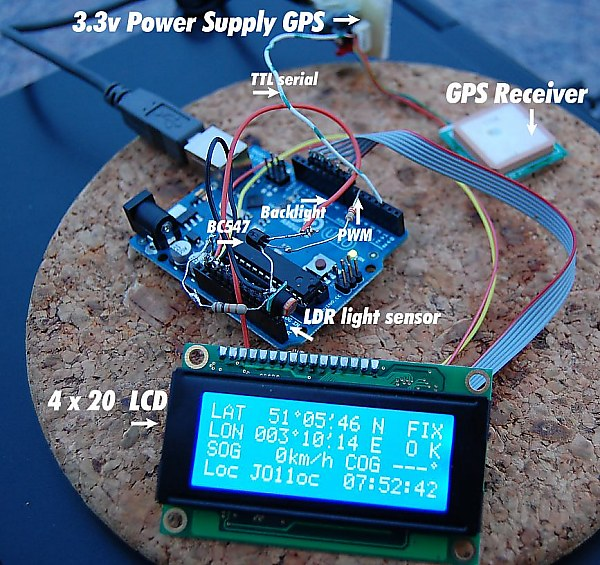The circuit in test - with ARDUINO UNO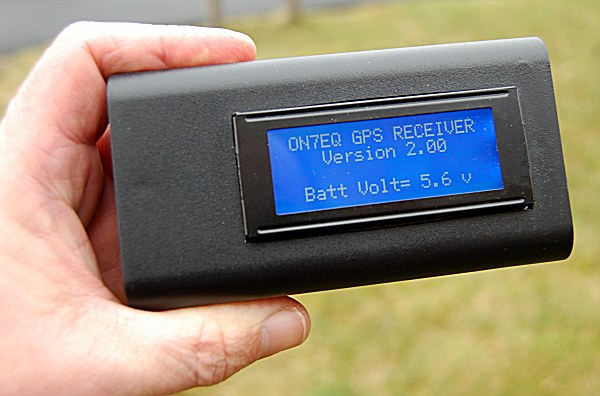The complete GPS finished ! Case size is  only 120 x 65 x 30 mm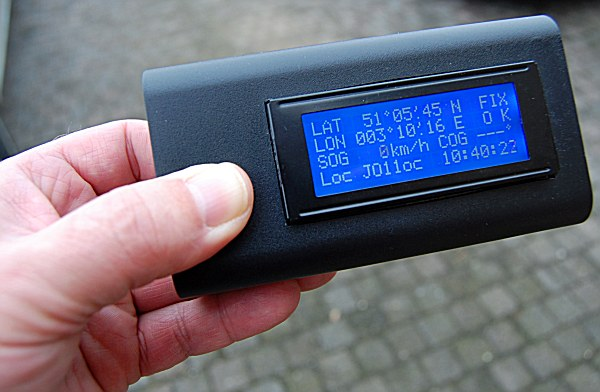Running on ARDUINO NANO board - Powered by 5x AAA NiMh batteries trough a low-drop 5v stabilizer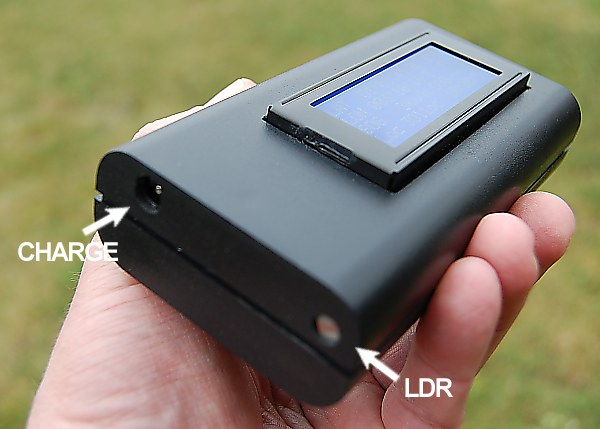On one side CHARGE / Power jack & LDR, on the other side of the case the power switch

Inside (click to enlarge)

This is the sketch  or download it here.   It was compiled with IDE version 0022.- IMPORTANT : please use the same or you might get errors when compiling !  You still can download previous versions from ARDUINO website .  You can find the library NewSoftSerial here. F5MMQ Patrick had some troubles working with TRIMBLE Copernicus II GPS, providing 5 digits accuracy after decimal point in LAT & LONG, and giving erratic readouts. Consequently the sketch was adapted (see comments).

Here is a rough schematic diagram of interconnections to the arduino board.

```

// A simple sketch to read GPS data and parse the \$GPRMC string

/*
*****************************
*    The GPRMC Sentence     *
*****************************

This sentence, known as the "Recommended Minimum" sentence, is the most common sentence transmitted by GPS devices.
This one sentence contains nearly everything a GPS application needs: latitude, longitude, speed, bearing, satellite-derived time,
fix status and magnetic variation.

Sentence Example
\$GPRMC,040302.663,A,3939.7,N,10506.6,W,0.27,358.86,200804,,*1A

Sentence Contents:
------------------
The GPRMC sentence consists of twelve comma-delimited words:

The Command Word:
\$GPRMC,040302.663,A,3939.7,N,10506.6,W,0.27,358.86,200804,,*1A
------
The command word indicates that the sentence is to be interpreted as a recommended minimum message.

Satellite-Derived Time:
\$GPRMC,040302.663,A,3939.7,N,10506.6,W,0.27,358.86,200804,,*1A
----------

GPS devices are able to calculate the current date and time using GPS satellites (and not the computer's own clock,
making it useful for synchronization). This word stores the current time, in UTC, in a compressed form "HHMMSS.XXX," where
HH represents hours,
MM represents minutes,
SS represents seconds,
and XXX represents milliseconds.
The above value represents 04:03:02.663 AM UTC.

Satellite Fix Status:
\$GPRMC,040302.663,A,3939.7,N,10506.6,W,0.27,358.86,200804,,*1A
-

When the signals of at least three GPS satellites become stable, the device can use the signals to calculate the current location.
The device is said to be "fixed" when calculations of the current location are taking place.
Similarly, the phrases "obtaining a fix" or "losing a fix" speak of situations where three signals become stable or obscured, respectively.

A value of "A" (for "active") indicates that a fix is currently obtained, whereas a value of "V" (for "inValid") indicates that a fix is not obtained.

Latitude Decimal Degrees:
\$GPRMC,040302.663,A,3939.7,N,10506.6,W,0.27,358.86,200804,,*1A
------

The latitude represents the current distance north or south of the equator. This word is in the format "HHMM.M" where
HH represents hours and
MM.M represents minutes.
A comma is implied after the second character. This value is used in conjunction with the longitude to mark a specific point
on Earth's surface. This sentence says that the current latitude is "39°39.7'N".

REMARK : Most GPS give precision in 4 digits after the decimal point, some give 5.  The sketch is to be adapted aacordingly (sse comments)

Latitude Hemisphere:
\$GPRMC,040302.663,A,3939.7,N,10506.6,W,0.27,358.86,200804,,*1A
-

This word indicates if the latitude is measuring a distance north or south of the equator.
A value of "N" indicates north and "S" indicates south. This sentence says that the current latitude is "39°39.7'N".

Longitude Decimal Degrees:
\$GPRMC,040302.663,A,3939.7,N,10506.6,W,0.27,358.86,200804,,*1A
-------

The longitude represents the current distance east or west of the Prime Meridian.
This word is in the format "HHHMM.M" where
HHH represents hours and
MM.M represents minutes.
A comma is implied after the third character. This value is used in conjunction with the latitude to mark a specific point on Earth's surface.
This sentence says that the current longitude is "105°06.6'W".

REMARK : Most GPS give precision in 4 digits after the decimal point, some give 5.  The sketch is to be adapted aacordingly (sse comments)

Longitude Hemisphere:
\$GPRMC,040302.663,A,3939.7,N,10506.6,W,0.27,358.86,200804,,*1A
-
This word indicates if the longitude is measuring a distance east or west of the Prime Meridian.
A value of "E" indicates east and "W" indicates west. This sentence says that the current longitude is "105°06.6'W".

Speed:
\$GPRMC,040302.663,A,3939.7,N,10506.6,W,0.27,358.86,200804,,*1A
----
This word indicates the current rate of travel over land, measured in knots.

Bearing:
\$GPRMC,040302.663,A,3939.7,N,10506.6,W,0.27,358.86,200804,,*1A
------

This word indicates the current direction of travel over, measured as an "azimuth."
An azimuth is a horizontal angle around the horizon measure in degrees between 0 and 360,

UTC Dat:
\$GPRMC,040302.663,A,3939.7,N,10506.6,W,0.27,358.86,200804,,*1A
------

GPS devices maintain their own date and time calculated from GPS satellite signals.
This makes GPS devices useful for clock synchronization since the date and time are independent of the local machine's internal clock.
This word contains two-digit numbers for days, followed by months and years. In the example above, the date is August (08) 20th (20), 2004 (04).
The two-digit year is added to 2000 to make a full year value.

The Checksum:
\$GPRMC,040302.663,A,3939.7,N,10506.6,W,0.27,358.86,200804,,*1A
---
*/

#include <LiquidCrystal.h>
// initialize the library with the numbers of the interface pins
LiquidCrystal lcd(7, 8, 9, 10, 11, 12);

/*
* LCD RS pin to digital pin 7
* LCD Enable pin to digital pin 8
* LCD D4 pin to digital pin 9
* LCD D5 pin to digital pin 10
* LCD D6 pin to digital pin 11
* LCD D7 pin to digital pin 12
* LCD R/W pin to ground
* GND to LCD VO pin (pin 3) (contrast)
*/

// include math functions
#include "math.h"

// Make sure to install newsoftserial from Mikal Hart
// http://arduiniana.org/libraries/NewSoftSerial/
#include <NewSoftSerial.h>

// Use pins 2 and 3 to talk to the GPS. 2 is the RX pin, 3 is the TX pin
NewSoftSerial mySerial =  NewSoftSerial(2, 3);

// voltage divider at A3  - select proper values so that voltage never exceeds 5v on Analog input !
// With R1 = 1k2 and R2 = 4k7, max input voltage = 25v

#define R1           (12)   // from GND to A3, express in 100R  (12 = 1200 Ohm)
#define R2           (47)   // from + power supply to A5, express in 100R  (47 = 4700 Ohm)

#define VoltSupplyMini (53) // minimum battery voltage expressed in 100mV (if lower, alarm is generated)
//                          // for low drop regulator like LM2940CT, minimum 5.5 v required

unsigned int SupplyVoltage = (0); // Power supply voltage
unsigned long BattCheck = 0;      // Battery Check Interval

// LCD specific characters 'degrees' and 'minutes'

byte degree  = {
B00100,
B01010,
B00100,
B00000,
B00000,
B00000,
B00000,
};

byte decminute  = {
B00100,
B00100,
B01000,
B00000,
B00000,
B00000,
B00100,
};

// Set the GPSRATE to the baud rate of the GPS module. Most are 4800
// but some are 38400 or other. Check the datasheet!
#define GPSRATE 4800

// Defines the PWM output pin for LCD backlight control
byte PWMOutPin = 5;

/* LDR Light sensor :

(+5v ) ---- (10k-Resister) -------|------- (LDR) ---- (GND)
|
A5 pin
*/

byte LCDlight  = 254; // LCD backlight value
byte Amblight  = 0 ;  // the ambiant light ( 0 = full light, 254 = dark)

// The buffer size that will hold a GPS sentence. They tend to be 80 characters long
// so 90 is plenty.
#define BUFFSIZ 90 // plenty big

// global variables
char buffer[BUFFSIZ];        // string buffer for the sentence
char *parseptr;              // a character pointer for parsing
char buffidx;                // an indexer into the buffer

//unsigned long StartTime = 0; // timing reference
unsigned long ParseTime = 0; // timing reference

// The time, date, location data, etc.
uint8_t hour, minute, second, year, month, date;
unsigned long latitude, longitude;
unsigned groundspeed, trackangle;
char latdir, longdir;
char fixstatus;
byte oldfixstatus = 0;  // previous fix status
unsigned long DisplayTime = 0;    // timer display refresh

char* FirstCharString[]={"A","B","C","D","E","F","G","H","I","J","K","L","M","N","O","P","Q","R"};
char* MidCharString[]={"0","1","2","3","4","5","6","7","8","9"};
char* LastCharString[]={"a","b","c","d","e","f","g","h","i","j","k","l","m","n","o","p","q","r","s","t","u","v","w","x"};

float scrap;  // variable for calculations
float loclat;  // variable for LOC calculations
float loclong;  // variable for LOC calculations

////////////////////    S E T U P ///////////////////////

void setup()
{

// set up the LCD's number of columns and rows:
lcd.begin(20, 4);
delay (200); // wait to allow LCD display to init

// Use the pin 13 LED as an indicator
pinMode(13, OUTPUT);

// Use the pin 3 LED as buzzer output
pinMode(3, OUTPUT);
digitalWrite(3, LOW);

// set PWM frequency on PWM output pin
setPwmFrequency(PWMOutPin, 256);
// For Pin 5&6 divisors are :  1 = 62.500 (=default) / 8 = 7.813 / 64 = 976 / 256 = 244 / 1024 = 61 Hz

// analogWrite(PWMOutPin)  LCD full brightness;
analogWrite(PWMOutPin, 254);

/////   A T T E N T I O N /////

// BECAUSE WE CHANGE PWM SOME TIMING FUNCTIONS - LIKE millis()
// ARE NO LONGER CORRECT
// WITH ABOVE PWM ADJUST, EFFECTIVE TIMES ARE ABOUT 3x LONGER THAN SET
// EXAMPLE :   delay(100)  will generate about 300ms delay

// connect to the GPS at the desired rate
mySerial.begin(GPSRATE);

// Print a message to the LCD.
lcd.setCursor(1, 0);
lcd.setCursor(4, 1);
lcd.print("Version 2.40");
// print supply voltage

SupplyVoltage = map(SupplyVoltage, 0,1023,0,(50*(R2+R1)/R1));

lcd.setCursor(2, 3);

delay (1000);

lcd.print("Batt Volt=");
if (SupplyVoltage < 100) {
lcd.print(" ");
}
if (SupplyVoltage < 10) {
lcd.print(" ");
}
lcd.print((SupplyVoltage/10), DEC);
lcd.print(".");
lcd.print((SupplyVoltage)%10, DEC);
lcd.print(" v");

delay (1000);

// create special characters
lcd.createChar(0, degree);
lcd.createChar(1, decminute);

PrintTemplate();

}

/////////////////  L O O P //////////////////////

void loop() {

uint32_t tmp;

Amblight = 255 - (analogRead (A5)/ 4);
if (Amblight < 20) Amblight = 10;

analogWrite(PWMOutPin, Amblight);

// Test Battery status

if ((millis() - BattCheck) > 20000) {  //every 60s check battery

BattCheck = millis();
SupplyVoltage = map(SupplyVoltage, 0,1023,0,(50*(R2+R1)/R1));

if  (SupplyVoltage <= VoltSupplyMini)   {  // We have a low voltage

lcd.clear();
lcd.setCursor(2, 1);
lcd.print("Batt Volt=");
if (SupplyVoltage < 100) {
lcd.print(" ");
}
if (SupplyVoltage < 10) {
lcd.print(" ");
}
lcd.print((SupplyVoltage/10), DEC);
lcd.print(".");
lcd.print((SupplyVoltage)%10, DEC);
lcd.print(" v");

lcd.setCursor(4, 3);
lcd.print("LOW BATTERY !");
digitalWrite(3, HIGH);  /// BEEP
delay(20);
digitalWrite(3, LOW);
delay(20);
digitalWrite(3, HIGH);
delay(20);
digitalWrite(3, LOW);

delay (1000);
PrintTemplate();
}
}

// End BattCheck

// check if \$GPRMC (global positioning fixed data)

if (strncmp(buffer, "\$GPRMC",6) == 0) {

digitalWrite(13, HIGH); /// DEBUG - valid string detected
delay (10);
digitalWrite(13, LOW);

// grab hhmmss time data
parseptr = buffer+7;
tmp = parsedecimal(parseptr);
hour = tmp / 10000;
minute = (tmp / 100) % 100;
second = tmp % 100;

// grab status
parseptr = strchr(parseptr, ',') + 1;
fixstatus = parseptr;
parseptr += 2;

// grab latitude data
latitude = parsedecimal(parseptr);
if (latitude != 0) {
latitude *= 10000; // if NMEA 4 digits precision after decimal point
//latitude *= 100000; if NMEA 5 digits precision after decimal point

parseptr = strchr(parseptr, '.')+1;
latitude += parsedecimal(parseptr);
}
parseptr = strchr(parseptr, ',') + 1;

// grab latitude N/S data
if (parseptr != ',') {
latdir = parseptr;
}

// grab longitude data
parseptr = strchr(parseptr, ',')+1;
longitude = parsedecimal(parseptr);
if (longitude != 0) {
longitude *= 10000;  // if NMEA 4 digits precision after decimal point
//longitude *= 100000; if NMEA 5 digits precision after decimal point
parseptr = strchr(parseptr, '.')+1;
longitude += parsedecimal(parseptr);
}
parseptr = strchr(parseptr, ',')+1;

// grab longitude E/W data
if (parseptr != ',') {
longdir = parseptr;
}

// grab groundspeed
parseptr = strchr(parseptr, ',')+1;
groundspeed = parsedecimal(parseptr);
groundspeed = groundspeed * 185; //kts to km/h
groundspeed = groundspeed / 100; //kts to km/h

// grab track angle
parseptr = strchr(parseptr, ',')+1;
trackangle = parsedecimal(parseptr);

// grab date
parseptr = strchr(parseptr, ',')+1;
tmp = parsedecimal(parseptr);
date = tmp / 10000;
month = (tmp / 100) % 100;
year = tmp % 100;

// update LCD

//latitude = latitude/10;   //if GPS NMEA 5 digits precision after DP
//longitude= longitude/10;  //if GPS NMEA 5 digits precision after DP

LCDprint();

} // end \$GPRMC NMEASTRING detected

}  ///////////// E N D    L O O P //////////////////

uint32_t parsedecimal(char *str) {
uint32_t d = 0;

while (str != 0) {
if ((str > '9') || (str < '0'))
return d;
d *= 10;
d += str - '0';
str++;
}
return d;
}

char c;

buffidx = 0; // start at beginning
while (1) {
if (millis() - ParseTime > 3000) NoGPS();
if (c == -1)
continue;
if (c == '\n')
continue;
if ((buffidx == BUFFSIZ-1) || (c == '\r')) {
buffer[buffidx] = 0;
return;
}
ParseTime = millis();
buffer[buffidx++]= c;
}
}

////////////////   GPS ERROR ////////////////////

void NoGPS () {

// analogWrite(PWMOutPin)  LCD full brightness;
analogWrite(PWMOutPin, 254);
//Print template ERROR MSG, no serial data detected
lcd.clear();
lcd.setCursor(4, 1);
lcd.print("NO GPS INPUT");
lcd.setCursor(1, 2);
lcd.print("CHECK  CONNECTIONS");
digitalWrite(3, HIGH);  /// BEEP
delay(50);
digitalWrite(3, LOW);
delay(1500);
ParseTime = millis();

PrintTemplate();
loop();
}

////////////////   PRINT TEMPLATE ////////////////////

void PrintTemplate () {

lcd.clear();
lcd.setCursor(0, 0);
lcd.print("LAT");
lcd.setCursor(5, 0);
lcd.print("--");
lcd.write(0);
lcd.print("--");
lcd.write(1);
lcd.print("--");

lcd.setCursor(0, 1);
lcd.print("LON");
lcd.setCursor(4, 1);
lcd.print("---");
lcd.write(0);
lcd.print("--");
lcd.write(1);
lcd.print("--");

lcd.setCursor(12, 2);
lcd.print("COG");
lcd.setCursor(16, 2);
lcd.print("---");
lcd.write(0);

lcd.setCursor(0, 2);
lcd.print("SOG");
lcd.setCursor(4, 2);
lcd.print("---");
lcd.print("km/h");

lcd.setCursor(0, 3);
lcd.print("Loc");
lcd.setCursor(4, 3);
lcd.print("------");

lcd.setCursor(12, 3);
lcd.print("HH");
lcd.setCursor(14, 3);
lcd.print(":");
lcd.print("MM");
lcd.setCursor(17, 3);
lcd.print(":");
lcd.print("SS");
}

////////////////   LCD    PRINT ////////////////////

void LCDprint () {

//NO FIX

if ((fixstatus == 'V') and (oldfixstatus == 1)) {
oldfixstatus = 0;
digitalWrite(3, HIGH);  /// BEEP alert : fix lost
delay(20);
digitalWrite(3, LOW);
}

if ((fixstatus == 'V')and (millis() - DisplayTime < 600)){
lcd.setCursor(17, 0);
lcd.print("N O");
lcd.setCursor(17, 1);
lcd.print("FIX");

}
if ((fixstatus == 'V')and (millis() - DisplayTime >= 600)){
lcd.setCursor(17, 0);
lcd.print("   ");
lcd.setCursor(17, 1);
lcd.print("   ");
DisplayTime = millis ();
}

// FIX OK

if (fixstatus == 'A') {
lcd.setCursor(17, 0);
lcd.print("FIX");
lcd.setCursor(17, 1);
lcd.print("O K");
oldfixstatus = 1;
}

// LCD LATITUDE

lcd.setCursor(5, 0);

if ((latitude/1000000)<10){
lcd.print("0");
}
lcd.print(latitude/1000000, DEC);
lcd.write(0);

if (((latitude/10000)%100)<10) {
lcd.print("0");
}

lcd.print((latitude/10000)%100, DEC);
lcd.write(1);
if ( ((latitude%10000)/100)< 10 ) {
lcd.print("0");
}
lcd.print((latitude%10000)/100, DEC);

lcd.setCursor(13, 0);
if (latdir == 'N')
lcd.print(" N ");
else if (latdir == 'S')
lcd.print(" S ");

// LCD LONGITUDE

lcd.setCursor(4, 1);

if ((longitude/1000000)<100) {
lcd.print("0");
}
if ((longitude/1000000)<10) {
lcd.print("0");
}
lcd.print(longitude/1000000, DEC);
lcd.write(0);

if (((longitude/10000)%100)<10) {
lcd.print("0");
}

lcd.print((longitude/10000)%100, DEC);
lcd.write(1);

if (((longitude%10000)/100)<10) {
lcd.print("0");
}
lcd.print((longitude%10000)/100, DEC);

lcd.setCursor(13, 1);
if (longdir == 'E')
lcd.print(" E ");
else if (longdir == 'W')
lcd.print(" W ");

// Print SOG

lcd.setCursor(4, 2);

if ((groundspeed)<100) {
lcd.print(" ");
}
if ((groundspeed)<10) {
lcd.print(" ");
}
lcd.print(groundspeed, DEC);

// COG print
lcd.setCursor(16, 2);

if (groundspeed <= 2) {
lcd.print("---");}

else {

if ((trackangle)<100) {
lcd.print("0");
}
if ((trackangle)<10) {
lcd.print("0");
}
lcd.print(trackangle, DEC);
}

// time

lcd.setCursor(12, 3);
if (hour < 10) {
lcd.print("0");
}
lcd.print(hour, DEC);

lcd.setCursor(15, 3);
if (minute < 10) {
lcd.print("0");
}
lcd.print(minute, DEC);

lcd.setCursor(18, 3);
if (second < 10) {
lcd.print("0");
}
lcd.print(second, DEC);

//

// Locator calculation //

/* debug - result must be BH52ig = Tahiti !

longdir = ('W');
latdir  = ('S');
longitude = 149176000;
latitude = 17438000;

*/
loclong = longitude;

loclong = 1000000 * int (loclong/1000000) +  ((loclong - 1000000 *int (loclong/1000000))/ 0.6);

if (longdir == 'E') loclong = (loclong) + 180000000;
if (longdir == 'W') loclong = 180000000 - (loclong);

loclat = latitude;

loclat = 1000000 * int (loclat/1000000) +  ((loclat - 1000000 * int (loclat/1000000))/ 0.6);

if (latdir == 'N')  loclat = loclat + 90000000;
if (latdir == 'S')  loclat = 90000000 - loclat;

lcd.setCursor(4, 3);

// First Character - longitude based (every 20° = 1 gridsq)

lcd.print(FirstCharString[int(loclong/20000000)]);

// Second Character - latitude based (every 10° = 1 gridsq)

lcd.print(FirstCharString[int(loclat/10000000)]);

// Third Character - longitude based (every 2° = 1 gridsq)

scrap = loclong  -   (20000000 * int (loclong/20000000));

//   lcd.print (scrap);  // D E B U G  field

lcd.print(MidCharString[int(scrap*10/20/1000000)]);

// Fourth Character - latitude based (every 1° = 1 gridsq)

scrap = loclat  -  (10000000 * int (loclat/10000000));
lcd.print(MidCharString[int(scrap/1000000)]);

// Fifth Character - longitude based (every 5' = 1 gridsq)

scrap = (loclong / 2000000)  -   (int (loclong/2000000));

lcd.print(LastCharString[int(scrap * 24)]);

// lcd.print (" ");    // D E B U G  field
// lcd.print (scrap,DEC);    // D E B U G  field
// lcd.print (loclong,DEC);    // D E B U G  field

// Sixth Character - longitude based (every 2.5' = 1 gridsq)

scrap = (loclat / 1000000)  -   (int (loclat/1000000));
lcd.print(LastCharString[int(scrap * 24)]);

} // end LCD display

//// Set PWM frequency
void setPwmFrequency(int pin, int divisor) {
byte mode;
if(pin == 5 || pin == 6 || pin == 9 || pin == 10) {
switch(divisor) {
case 1: mode = 0x01; break;
case 8: mode = 0x02; break;
case 64: mode = 0x03; break;
case 256: mode = 0x04; break;
case 1024: mode = 0x05; break;
default: return;
}
if(pin == 5 || pin == 6) {
TCCR0B = TCCR0B & 0b11111000 | mode;
} else {
TCCR1B = TCCR1B & 0b11111000 | mode;
}
} else if(pin == 3 || pin == 11) {
switch(divisor) {
case 1: mode = 0x01; break;
case 8: mode = 0x02; break;
case 32: mode = 0x03; break;
case 64: mode = 0x04; break;
case 128: mode = 0x05; break;
case 256: mode = 0x06; break;
case 1024: mode = 0x7; break;
default: return;
}
TCCR2B = TCCR2B & 0b11111000 | mode;
}
}
//// end of PWM set

```